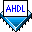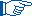## Using Numbers

 See AlsoNumbers are used to specify constant values in Boolean expressions and equations, arithmetic expressions, and parameter values. AHDL supports all combinations of decimal, binary, octal, and hexadecimal numbers.

The decode1.tdf file shown below is an address decoder that generates an active-high chip enable when the address is 370 Hex.

```SUBDESIGN decode1
(
chip_enable    : OUTPUT;
)
BEGIN
END;
```

In this sample file, the decimal numbers `15` and `0` are used to specify bits of the address bus. The hexadecimal number `H"0370"` specifies the address that is decoded.

The following rules apply to numbers:

• In a Text Design File (.tdf) that uses Boolean equations to tie unused single- or dual-range group inputs to `VCC` or `GND`, the Compiler requires unambiguous numeric values. For example, the following equation contains ambiguous values:

`a[7..0] = (H"2", H"3")`

In this equation, the Compiler converts the non-binary numbers to the minimum number of binary bits and then sign-extends them. The Compiler therefore yields a binary value of `B"0000 10 11"`, not `B"0010 0011"`. Similarly, the following equation yields an error indicating that there is an unequal number of bits on the two sides of the equation:

`a[7..0] = (B"0010", H"3")`

The Compiler interprets the value of the expression as `B"001011"`, not `B"00100011"`. Altera® recommends using binary numbers to avoid ambiguity in these types of expressions.

• In contrast to all versions of the Quartus® software previous to version 2000.09, this version can contain AHDL numbers with more than 32 bits. This change results in the following rules:

• A number that is not a binary number has different sizes depending on the context in which it is located (for example, depending on whether it is in a Boolean equation or an arithmetic expression). For example, depending on its location, `H"2"` can be represented with 2, 3, or 4 bits. (However, as described above, the Compiler always converts non-binary numbers to the minimum number of binary bits during compilation.)

• A binary, octal, and hexadecimal number that has exactly 32 bits is never negative when its MSB is `1`. For example, `H"FFFF0000"` is not a negative number.For backward compatibility with versions of the Quartus software previous to version 2000.09, you can limit AHDL numbers to 32 bits by turning on the Limit AHDL Integers to 32 Bits logic option for a design entity.